# PYTHON 基础笔记

## 1.字符串

### 1.1输出

message = "Hello World"
a = 1
b = a/2
print(message)
print(a,b)
print(message + " Again")
print(message + str(a))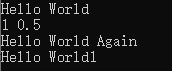a = 1
b = 2
print(a,b,end = '')##输出1空格2


### 1.2字符修改

a.lower()将字符串a转化成全部小写字母,但a不变
a.title()将字符串a转化成首字母大写形式
a.upper()将字符串a转化成全部大写字母
a.lstrip()去除a首部的空白
a.rstrip()去除a末尾的空白
a.strip()去除a首尾的空白
a.split()将a以分隔符为分隔，返回形成的列表
# -*- coding:utf-8 -*-
message = " a BcDE fgh "
print(message.lower())##小写
print(message.upper())##大写
print(message.title())##首字母大写
print(message)##原来变量未变

print(message.rstrip())##去除末尾空白
print(message.lstrip())##去除首部空白
print(message.strip())##去除两端空白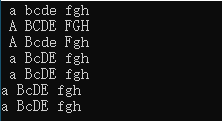2)#coding:utf-8
3)#coding=utf-8

## 2.列表

### 2.1基本

a=[‘a’,‘b’,1,2]a是个列表，含有a,b,1,2四个元素
a[i]访问列表中第i个元素，下标从零开始算，i可以为负数，意味着倒数，如-1表示最后一个
a[i] = ‘c’将列表第i个元素修改为c，下标不能超过界限
a.append(‘d’)在列表末尾添加元素d
a.insert(i,‘e’)在列表第i处添加元素e
del a[i]删除列表中第i个元素
a.remove(‘b’)删除列表中为b的元素
a.pop(i)弹出列表第i个元素，可以将其赋值给其他变量，i默认-1
set(a)将列表中重复元素临时删去只剩下一个，列表不变
#coding: utf-8
a = ['a','b',1,2]
print(a)
a = 'A'
print(a)#显示修改a的结果
a.append(3)
print(a)#显示添加后结果
del a
print(a)#显示删除a的结果
temp = a.pop()
print(a)
print(temp)#显示弹出后a的结果及弹出值
a.remove('b')
print(a)#显示删除指定值的结果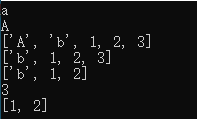a.remove()删除的值一定在列表中有，否则会报错

### 2.2组织列表

sorted(a)对a临时排序，可传入reverse = True反序。不改变列表.下面两种均会改变
a.sort()将列表按英文字母大小写排序，可传入reverse = True
a.reverse()列表反向
len(a)返回列表长度
#coding: utf-8
a = ['b','c','d','a']
print(sorted(a))
print(a)#sorted(a)未改变列表
a.sort()
print(a)#改变列表
a.reverse()
print(a)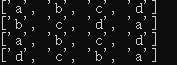### 2.3遍历列表

#coding: utf-8
a = ['b','c','d','a']
for a_  in a:
print(a_.upper())
print(a_)


### 2.4创建数值列表

#coding: utf-8
for i in range(1,5):
print(i)
print('')
for i in range(1,5,2):
print(i)
print(range(1,5))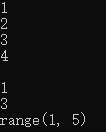#coding: utf-8
a = list(range(1,5))
print(a)

b = []
for c in range(1,5):
c = c**2
b.append(c)
print(b)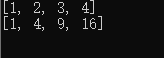a =[i**2 for i in range(1,5)]
print(a)


max(a)返回列表a中最大的数值
min(a)返回列表a中最小的数值
sum(a)返回列表a中各数值求和

### 2.5切片

#coding: utf-8
a = [i**2 for i in range(1,5)]
for a_ in a:
print(a_,end=' ')
b = a
for i in range(0,4):
b[i]=b[i]+1
print()
print(a)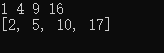#coding: utf-8
a = [i**2 for i in range(1,5)]
for a_ in a:
print(a_,end=' ')
b = a[1:3]#（从0开始）将a的第1个和第2个元素形成的列表赋值给b
for i in range(0,len(b)):
b[i]=b[i]+1
print()
print(a)
print(b)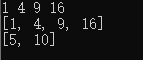## 3.if语句

if语句同C一样可用于条件判断，并且条件表达式不一定要加括号。但表达式中不能像C一样将某个变量赋值。if 还可以判断列表是否含有元素,if 5 in a或者if 5 not in a
if语句的形式有以下

1.if a > b:
语句
2.if a < b:
语句
else:
语句
3.if a < b:
语句
elif a !=b:

#coding: utf-8
a = 1
b= 2
c = [1,2,3,4,5]
if a > b:
print("a>b")
elif (a < b):
print("a<b")
if (a+10 not in c):
print(c)
if 5 in c:
print ("c has 5" )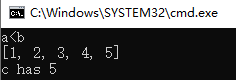## 4.字典

a={‘a’:1}建立字典包含a-1这个键值对
a={‘b’:2}如果字典a中存在’b’这个键，则修改b对应的值为2。如果没有则添加映射
a访问a中1为键对应的值
del a[‘b’]删除字典a中键b以及对应的值
a.items()返回键值对
a.keys()返回键
a.values()返回值
#coding: utf-8
#coding: utf-8
test = {'a':[1,2,3],'b':4,'c':5}
print(test.keys())
for key,value in test.items():
print(key +'---'+ str(value))
print()
del test['a']
for value in test.values():
print(value)
print(list(test.items()))
print(list(test.values()))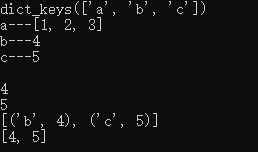## 5.用户输入

message = ''
print(message)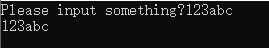## 6.While循环

while循环与C类似，如果后面表达式为1则执行循环，为0则不执行。不要忘记冒号和缩进。同样可以while 'a' in test:来判断a是否在列表中。break可用来退出当前循环，continue可退出当前循环执行下一次循环。

#coding: utf-8
for a in range(1,10):
if(a == 5):
continue
print(a)
print()
for a in range(1,10):
if(a == 5):
break;
print(a)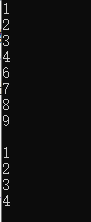02-022万+
04-2246